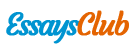# Biological Models with Time Delay Differential Equations

Autor:   •  January 9, 2019  •  647 Words (3 Pages)  •  155 Views

Page 1 of 3

...

[pic 43]

And, [pic 44]

Solving (16),(17) and (18) the equilibrium point of the system is [pic 45].

Positivity test:

Now we will prove that all the variable that in the system model equations are positive.

If, [pic 46] then solution of [pic 47] and [pic 48] of the model system of equations (13-15) are positive.

Proof:

To prove this theorem we get from the equation (13)

[pic 49]; Neglecting the term where o is not include.

[pic 50]

Integrating factor [pic 51]

Now multiplying the I.F on both side of (19) we get

[pic 52]

[pic 53]

Integrating both side we get,

[pic 54]

Where c is a constant.

[pic 55]

For the value of c, we applying the initial condition when [pic 56] the,

[pic 57] we get [pic 58]

Putting the value in (20) we get

[pic 59]

Since,[pic 60], hence [pic 61] if [pic 62] and therefore [pic 63] for all [pic 64]

Again, we get from the equation (14)

[pic 65]

Neglecting the terms where p is not include.

[pic 66]

[pic 67]

So we can write from (22)

I.F [pic 68]

[pic 69]

Now, multiplying the I.F on the both side of (22)

[pic 70]

Integrating both side ,we get

[pic 71]

Where c is integer constant.

The initial condition when [pic 72], then [pic 73]

Hence, [pic 74]

Putting this in (23)

[pic 75]

Since, [pic 76]and [pic 77]

Therefore, [pic 78]for all [pic 79]

And from equation (15) we can wrire,

[pic 80]

Neglecting the terms which is not include z.

[pic 81]

I.F= [pic 82]

Multiplying the IF into (24)

[pic 83]

[pic 84]

Integrating, both side

[pic 85]

C is a integer constant

When [pic 86] then, [pic 87]

Hence, from (25) we get

[pic 88]

[pic 89]

Since, [pic 90] and [pic 91].therefore [pic 92] for all [pic 93]

Finally, we get all the variable that in the system model equations are positive.

...